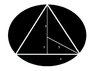# Applied Optimization Problem

• Willowz

## Homework Statement

Find the volume of the largest right circular cone that can be inscribed in a sphere of radius 3.

V=π*r^2*h/3
A=πr^2 + πrl

## The Attempt at a Solution

I did multiple things that I'm not sure are correct. I took the derivative for the volume with the value of h set to (9-x^2)^(1/2). The derivative I got was;
3π/2 * (9-x^2)^(-1/2) * (-2x)

Not really sure what to do. Think I need a hint on the steps that need to be taken. Picture of problem attached.

#### Attachments

•problem.jpg
17.1 KB · Views: 387
Last edited:
In your diagram, I would replace "y" with "h - 3". Next, find x in terms of h. Then substitute into the volume of a right circular cone
$V = \frac{1}{3}\pi r^2 h$
(with r = x here) and you'll have a function with one variable, h. Now find dV/dh and go on from there.

## Homework Statement

Find the volume of the largest right circular cone that can be inscribed in a sphere of radius 3.

V=π*r^2*h/3
A=πr^2 + πrl

## The Attempt at a Solution

I did multiple things that I'm not sure are correct. I took the derivative for the volume with the value of h set to (9-x^2)^(1/2). The derivative I got was;
3π/2 * (9-x^2)^(-1/2) * (-2x)

Not really sure what to do. Think I need a hint on the steps that need to be taken. Picture of problem attached.

Write out your expression for V in terms of x. I got a different derivative dV/dx than yours.

RGV